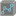• # Solution works in local editor only

## Question related to missionGraphical Key

Hello everybody! I hope you can help figure out the reason of the following problem.

This solution works in my local editor (PyCharm 2019.1.3, Win10, 64-bit) but not in the online editor:

```from itertools import product

def valid_neighbours(max_length, start, path, grid_dim):
row, col = start
vertices = set()
for i, j in product([-1, 0, 1], [-1, 0, 1]):
if any([row+i<0, col+j<0]) or any([row+i>grid_dim, col+j>grid_dim]):
continue
if grid_dim - min([row+i, col+j]) + 1 <= max_length - len(path):
return vertices

def dfs_g_key(grid, start, goal, max_length, path=None):
if path == None:
path = set([start])
if start == goal:
yield path
vertices = valid_neighbours(max_length, start, path, len(grid)-1) - path
for vertex in vertices:
yield from dfs_g_key(grid, vertex, goal, max_length, path | set([vertex]))

def g_key(grid, N):
if N == len(grid) ** 2:
return sum([sum(x) for x in grid])

max_sum = float("-inf")
for path in dfs_g_key(grid, (0, 0), (len(grid)-1, len(grid)-1), N):
max_sum = max(max_sum, sum([grid[i][j] for i, j in path]))
return max_sum
```

Once I change it to this one, it works in both, local and online editors:

```from itertools import product

def valid_neighbours(max_length, start, path, grid_dim):
row, col = start
vertices = set()
for i, j in product([-1, 0, 1], [-1, 0, 1]):
if any([row+i<0, col+j<0]) or any([row+i>grid_dim, col+j>grid_dim]):
continue
if grid_dim - min([row+i, col+j]) + 1 <= max_length - len(path):
return vertices

def dfs_g_key(grid, start, goal, max_length, path=None):
if path == None:
path = set([start])
if start == goal:
yield path
vertices = valid_neighbours(max_length, start, path, len(grid)-1) - path
if not vertices:
yield None
for vertex in vertices:
if vertex == goal:
yield path | set([vertex])
else:
yield from dfs_g_key(grid, vertex, goal, max_length, path | set([vertex]))

def g_key(grid, N):
if N == len(grid) ** 2:
return sum([sum(x) for x in grid])

max_sum = float("-inf")
for path in dfs_g_key(grid, (0, 0), (len(grid)-1, len(grid)-1), N):
if path is None:
continue
max_sum = max(max_sum, sum([grid[i][j] for i, j in path]))
return max_sum
```